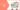Dark Light

# How Many Years Will It Take To Double Your Money? Read To Know More!# With this magic rule, you may just become smart enough to manage your own money!

In personal finance, if you divide the number 72 by the rate of interest, you get to know the number of years it will take for you to double the money.
Example: If the rate of interest is 9%, simply divide the number 72 by 9% and the answer is 8. Thus it will take 8 years to double your money if you invest at the rate of interest of 9% p.a.

### INTEREST:

We can use this rule in reverse to know the rate of interest needed to double your money to achieve your set goal.
Eg: If you have 250k today and you need 500k in 5 years. Just divide the number 72 by 5, the answer is 14.41%. Thus you need a type of investment avenue, where you earn at least 14.41% p.a. as the rate of interest/returns to double your investment amount in 5 years.

### INFLATION:

This ‘Rule 72’ helps you to understand about inflation also. It helps you to calculate the amount of time it will take for inflation to make the real value of money half. Let’s say present inflation is 5.5%. When you divide 72 by 5.5% the answer is 13.09 years. That is to say, if you have 100k in your kitty today, it would take around 13.09 years for the value of the money to be halved.

### HOW DOES THE FORMULA WORKS?

The Rule of 72 is a great mental math shortcut to estimate the effect of any growth rate, from quick financial calculations to population estimates. Here’s the formula:

`Years to double = 72 / Interest Rate`

This formula is useful for financial estimates and understanding the nature of compound interest. Examples:

• At 6% interest, your money takes 72/6 or 12 years to double.
• To double your money in 10 years, get an interest rate of 72/10 or 7.2%.
• If your country’s GDP grows at 3% a year, the economy doubles in 72/3 or 24 years.
• If your growth slips to 2%, it will double in 36 years. If growth increases to 4%, the economy doubles in 18 years. Given the speed at which technology develops, shaving years off your growth time could be very important.

You can also use the rule of 72 for expenses like inflation or interest:

• If inflation rates go from 2% to 3%, your money will lose half its value in 24 years instead of 36.
• If college tuition increases at 5% per year (which is faster than inflation), tuition costs will double in 72/5 or about 14.4 years. If you pay 15% interest on your credit cards, the amount you owe will double in only 72/15 or 4.8 years!

The rule of 72 shows why a “small” 1% difference in inflation or GDP expansion has a huge effect in forecasting models.

By the way, the Rule of 72 applies to anything that grows, including population. Can you see why a population growth rate of 3% vs 2% could be a huge problem for planning? Instead of needing to double your capacity in 36 years, you only have 24. Twelve years were shaved off your schedule with one percentage point.

Hope, the Rule Of 72 that we explained you here, definitely help you in your day to day investments and other finance-related activities.

Scroll To Top